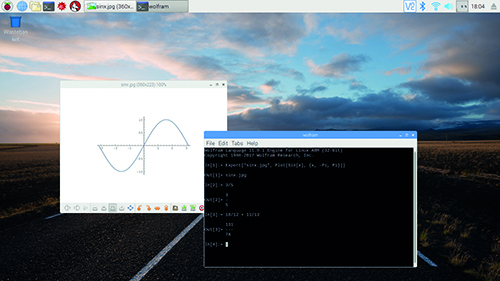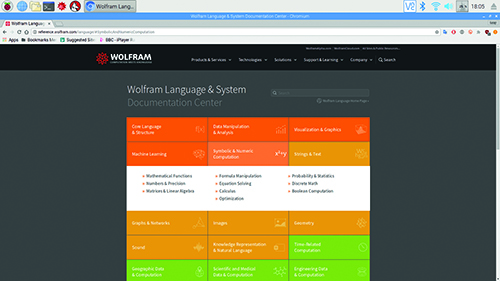Learn to use Wolfram Language

By Lucy Hattersley. Posted

All computing problems are, at heart, maths problems. And you can solve many problems using a programming language like Wolfram.

A Raspberry Pi computer is an ideal companion for those looking to learn more about mathematics. Maths is all about problem-solving, and learning the language of mathematics can help you solve all kinds of real-world problems.

Computers take a lot of the grunt work out of maths, and help you focus on the underlying mechanics (rather than grinding at the problems). Or, if you are trying to learn maths techniques, you can use a computer to check your answers.Using Wolfram Language in Raspbian

Thanks to a special deal between Raspberry Pi and Wolfram Research, the standard operating system Raspbian comes pre-installed with software called Wolfram Mathematica and Wolfram Language.

The great thing about Wolfram Language and Mathematica is that you can perform high-end data calculations that make use of Wolfram’s Knowledge Base (which lets you tap into thousands of data points: things like global rainfall levels, mobile phone ownership, and sports data).

Mathematica’s graphical interface can be confusing, so we think it’s better to start with the Wolfram Language in the command line.

Wolfram Language has lots of advantages over using maths in other environments or languages, such as Python. Wolfram Language is deeply focused on maths. It is much easier for working with rational numbers and radians (which aren’t converted to decimal and degrees by default).

In this tutorial we’re going to cover some of the starting points for Wolfram Language on your Raspberry Pi. With it you’ll be able to use Wolfram Language instead of a calculator, and use it to explore mathematical concepts.

Get started with Wolfram Language

Start by clicking on the Wolfram icon in the taskbar or via Menu > Programming > Wolfram.

This opens a terminal window displaying the following command prompt:

In:=

You can also access this prompt from the command line by entering ‘Wolfram’.

Performing simple maths is easy enough. Just enter your maths term followed by ENTER:

2 + 2

And you’ll see:

Out= 4

In:=

You can now enter another command, such as:

2100

And you’ll see:

Out= 1267650600228229401496703205376

This is in decimal notation, not scientific notation as you’d get in Python.

As with other programming languages, you can store items as variables and use them:

x = 2+2
y = 2100
x * y

You can flip back and forth through previous input lines using the Up and Down arrows. You can also repeat previous output lines using the Out[] function with the line number. Such as:

Out
Out
Out * Out

Or use the % symbol:

%1
%2
%1 * %2

Using just % gets the last output.

Functions in Wolfram Language

Functions form a vital part of Mathematica. In fact, everything – including simple mathematical expressions such as 2+2 – is converted to a function. Functions always start with a capital letter, while input values are contained by square brackets:

Plus[2,2]
Times[2,10]
Power[2,100]
Divide[100,4]

Even setting variables is actually a function. And functions can be nested inside one another. These three commands all mean the same thing:

x = 2+2
Set[x,2+2]
Set[x,Plus[2,2]]

There is also a clear function, Unset[x], if you want to get rid of variables.

This doesn’t mean you’re supposed to translate every expression into its functional equivalent. But some expressions just aren’t that easy to do manually. So the Sqrt[] function is required:

Sqrt

Getting rational with Wolfram Language

One of the real joys of using Wolfram is its handling of rational numbers. Enter 1/2 in Python and you’ll get 0 or 0.5 (depending on whether you’re using Python 2 or 3). Enter 1/2 in Wolfram Language and you’ll get a satisfying

1
-
2

Rational numbers are also reduced in form to the smallest denominator. Enter 3/15 and you’ll get:

1
-
5

The FullForm function for a rational number is Rational[1,5] if you want to use it. You can combine rationals with integers, such as:

2 * 1/5

2
-
5

If you’d rather get a number outputted as a decimal, the easiest way is to add a decimal point to one of the numbers in the rational.

2* 1./5

0.4

Alternatively, you can surround it in an N function.

N[1/3]

0.333333

By default, N returns numbers to six-digit precision. A second value in N can be used to adjust the precision:

N[1/3, 10]

0.3333333333

You can convert numbers to a nearby rational with the smallest denominator using the Rationalize function

Rationalize[0.5]

1
-
2

Lists in Wolfram Language

Now that you’ve got the hang of basic mathematical functions, you can start to use Wolfram Language as a programming tool.
Lists form a central part of Wolfram Language and can be used to group together items. Lists are placed between curly braces {} and can be created manually:

{1, 3, 5}

Or by using the List function:

List[1,3,5]
{1, 3, 5}

And you can generate lists using the Range command:

Range
{1, 2, 3, 4, 5}

Use two numbers to provide a start and stop point:

Range[5,10]
{5, 6, 7, 8, 9, 10}

And three numbers to use a start, stop, and step:

Range[2,8,2]
{2, 4, 6, 8}

Visualisation and export in Wolfram Language

Wolfram Language has a host of tools for visualising data. Plot is a common one used to plot the output of functions. Generally it’s best to switch to Mathematica if you want to get a visual view of your function, but it is possible to export graphics from the command line to an image using the Export function.

Take the Plot function which graphs the Sin function of x against a list of x values from -Pi to Pi.

Plot[Sin[x],{x, -Pi, Pi}]

In Mathematica you’ll get a lovely visualisation, while in Wolfram Language you just get:

-Graphics-

Surround the function in an Export function with the first value a file name and the second the function:

Export["sinx.jpg",Plot[Sin[x],{x, -Pi, Pi}]]

It will be saved in your /home/pi
directory by default. To view the graph, open a Terminal and enter:

xdg-open sinx.jpg

Create functions in Wolfram Language

You can create your own functions inside Wolfram by entering a name for your function with square brackets. Then placing a variable placeholder, which is a letter followed by an underscore, such as x_, inside the brackets:

User-generated functions are lower-case by convention. The name is followed by a colon and equals sign:

After the equals sign we add the expression, which uses the same variable without the underscore:

To use the function, we simply use its name and add variables into the brackets (as we would any regular function).

4

6

With all of these features and functions, and the ability to work with integers, rational, and real numbers (plus a wide range of other numbers), Wolfram Language is a powerful tool. We’ve only scratched the surface of what you can do with it here, and it is packed with Boolean operators and procedural processes (such as for and while loops).

Hopefully you’ve got enough here to find Wolfram Language less daunting. Visit reference.wolfram.com for the online documentation. Be sure to use Wolfram Language next time you’re working with maths.Wolfram Language Documentation

You can find a list of all Wolfram functions, commands, and techniques in the Wolfram Language & System Documentation Center.